• 运动学（Kinematics） 1.基础部分（叉乘相关知识） 2. 推导坐标系变换的公式
运动学（Kinematics）

1.基础部分（叉乘相关知识）

2. 推导坐标系变换的公式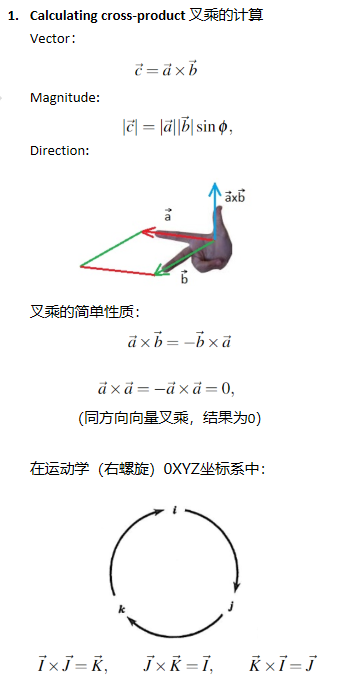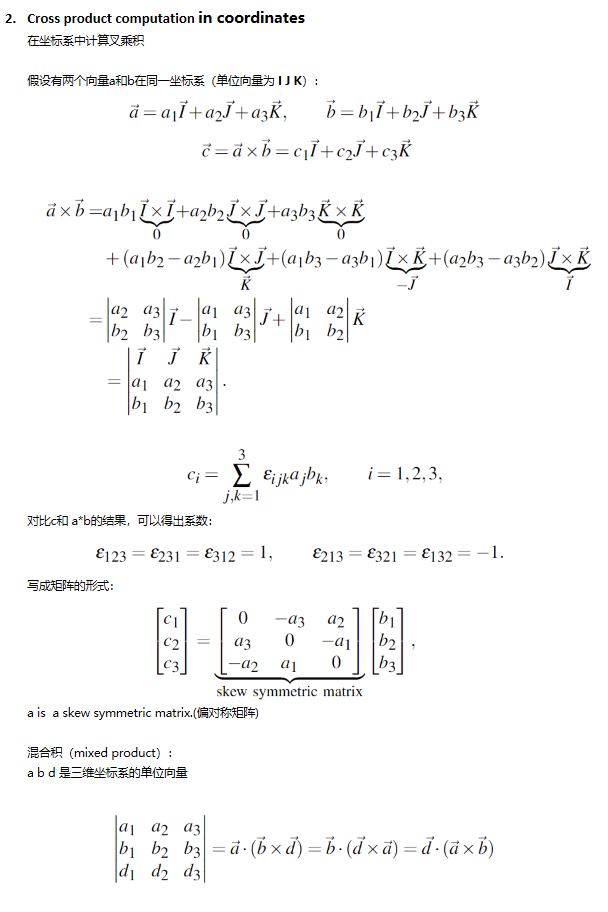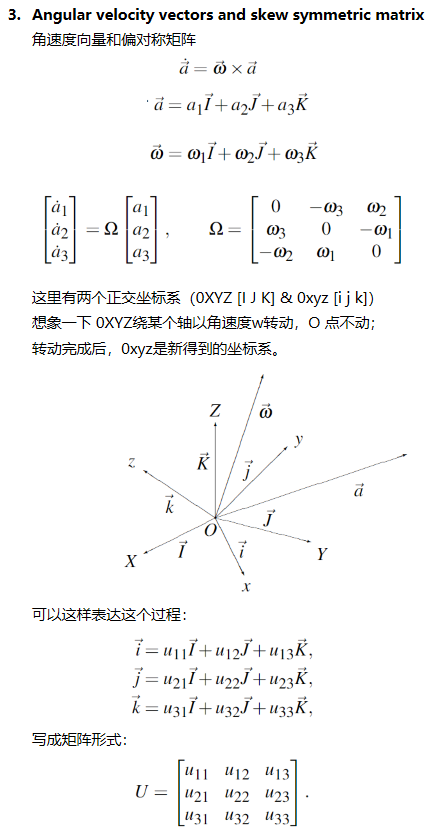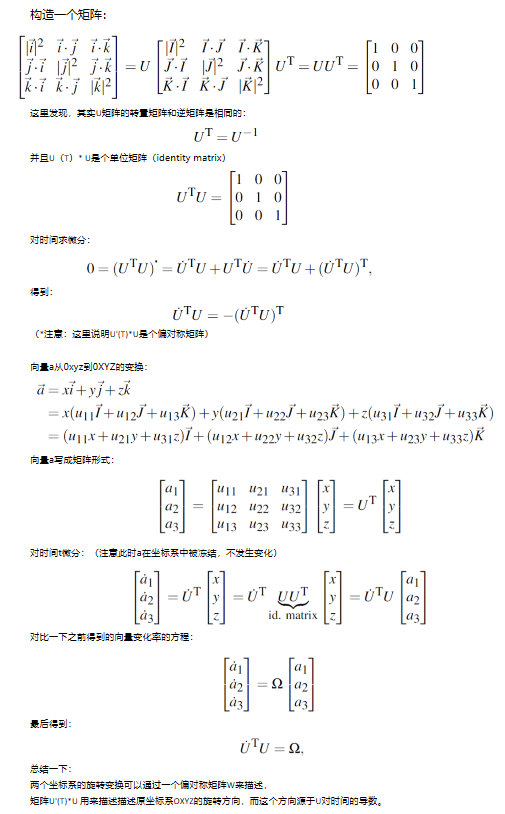展开全文• 设两向量分别为 α 和 β， ...　通过公式我们可以发现，两个向量的数量就是一个数量。 　数量又称为点或者内。 　ex: 在直角坐标系 {O; i, j, k} 中，设 α = (a1, a2, a3), β = (b1, b2, b3), 　...

设两向量分别为 α 和 β，
数量积
α • β = |α| |β| cosθ 　　（θ 为向量 α 和 β 的夹角）
通过公式我们可以发现，两个向量的数量积就是一个数量。
数量积又称为点积或者内积。
ex: 在直角坐标系 {O; i, j, k} 中，设 α = (a1, a2, a3), β = (b1, b2, b3),
α • β = (a1i + a2j + a3k) • (b1i + b2j + b3k) = a1b1 + a2b2 + a3b3
即两向量的数量积之和等于它们对应坐标的乘积之和。

向量积
向量积是一个向量，通常表示为 α χ β ，
1. 它的模(即长度)为 |α χ β| = |α| |β| sinθ 　　（θ 为向量 α 和 β 的夹角）
2. 方向垂直于向量 α 和 β，且 (α, β, α χ β) 构成右手系。
向量积又称为叉积和外积。
ex: 在直角坐标系 {O; i, j, k} 中，设 α = (a1, a2, a3), β = (b1, b2, b3),
α χ β = (a1i + a2j + a3k) χ (b1i + b2j + b3k)
= (a2b3 - a3b2)i - (a1b3 - a3b1)j + (a1b2 - a2b1)k
行列式表示为即混合积
向量α 与 β 的向量积，再与向量 γ 作数量积，其结果为一个数量，称这个数量为
三向量的 α, β, γ 的混合积，记为 (α, β, γ), 即
(α, β, γ) = (α χ β) • γ
1. 三向量共面的充要条件为 (α, β, γ) = 0
2. （空间向量基本定理）任意给定空间中三个不共面向量 α, β, γ，则空间中任一
向量 ν 可以用 α, β, γ 唯一线性表示，即存在唯一一组实数 x, y, z 使
ν = xα + yβ + zγ
ex: 空间向量运算
在直角坐标系 {O; i, j, k} 中，设 α = (a1, a2, a3), β = (b1, b2, b3),
γ = (c1, c2, c3)即转载于:https://www.cnblogs.com/openxyz/p/6789184.html
展开全文• 六维空间向量表示法目录1、运动和力2、空间速度和空间力3、加法运算和模乘4、点积(内积)5、坐标变换6、叉积(向量积，外积)7、微分8、加速度9、空间动量10、空间惯量11、空间运动方程 目前对机器人动力学还没有一套...
六维空间向量表示法目录1、运动和力2、空间速度和空间力3、加法运算和模乘4、点积(内积)5、坐标变换6、叉积(向量积，外积)7、微分8、加速度9、空间动量10、空间惯量11、空间运动方程
目前对机器人动力学还没有一套标准的表示法，比如有3D向量、齐次矩阵、六维空间向量等。其中最有效的是六维空间向量（ Spatial Vector Notation），本文是我看《Handbook of robotics》的笔记，其中看起来弯弯曲曲的字母$v f$表示3维向量，看起来直直的v f 表示六维空间向量。
1、运动和力
为了区分刚体的运动（motion）和施加在刚体上的力（force），分别用运动六维向量空间$M^6$和力六维向量空间$F^6$来表示。两个空间及其各自的基向量如下图所示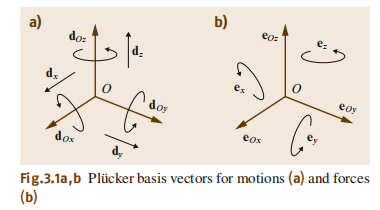两个空间共有12个基向量，6个用来描述运动，6个用来描述力。
在运动六维空间，对于一个坐标系$O_{xyz}$，三个描述分别绕坐标轴$O_x$，$O_y$，$O_z$进行旋转的基向量$d_{Ox}$，$d_{Oy}$，$d_{Oz}$；三个描述分别沿着坐标轴$O_x$，$O_y$，$O_z$进行平移的基向量$d_{x}$，$d_{y}$，$d_{z}$。
在力六维空间，对于一个坐标系$O_{xyz}$，三个描述分别绕坐标轴$O_x$，$O_y$，$O_z$进行旋转的基向量$e_{Ox}$，$e_{Oy}$，$e_{Oz}$；三个描述分别沿着坐标轴$O_x$，$O_y$，$O_z$进行平移的基向量$e_{x}$，$e_{y}$，$e_{z}$。
2、空间速度和空间力
任意给定一个参考点$O$，刚体的速度可以用一对3维向量来表示：沿三个轴的线速度$v=(v_{Ox},v_{Oy},v_{Oz})$、绕三个轴旋转的角速度$w=(w_x,w_y,w_z)$。那么该运动的六维空间表示法为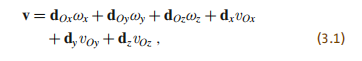相应的其普吕克坐标表示为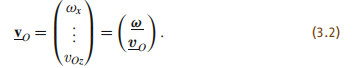关于力是相似的。刚体受到力$f$和扭矩$n_O$的作用，则该受力情况的六维空间向量表示法为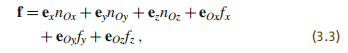相应的其普吕克坐标表示为3、加法运算和模乘
如果刚体同时受到两个力f$_1$和f$_2$的作用，那么这两个力的合力为f$_1$+f$_2$；
如果刚体1的速度为v$_1$，刚体2的速度为v$_2$，那么刚体2相对于刚体1的运动速度为v$_2$-v$_1$；
如果刚体受到力f$_1$的作用，其大小为1N，那个$α$f$_1$表示在该方向上大小为$α$N的力。
4、点积(内积)
六维空间向量的点积定义为该物体的运动m∈M$^6$和力f∈F$^6$的点积——f·m或者m·f。
m·m和f·f是无定义的。
如果m和f是用同一坐标系来表示的，那么m·f=m$^T$f。
5、坐标变换
定义$A$和$B$是两个坐标系，在$A$坐标系下的运动和力分别表示为m$_A$和f$_A$；在$B$坐标系下的运动和力分别表示为m$_B$和f$_B$。
那么从$A$坐标系下的运动变换到$B$坐标系下的方程为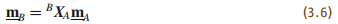相应的力的变换为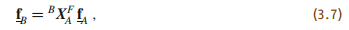其中$^BX_A$和$^BX_A^F$分别是从坐标系$A$到坐标系$B$的运动变换矩阵和力变换矩阵。并且二者满足如下关系，也就是从力变换矩阵到运动变换矩阵的变换(或者反过来)是先求逆再转置(求逆和转置可交换)。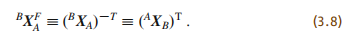假设坐标系$A$相对于坐标系$B$的位置向量为$^Bp_A$，旋转变换矩阵为$^BR_A$，那么$^BX_A$可以表示为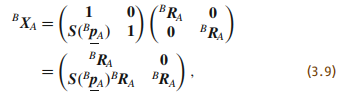其逆矩阵为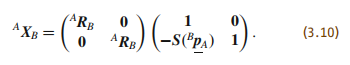其中$S(p)$为$p$的斜对称矩阵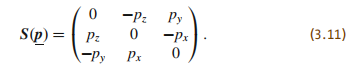6、叉积(向量积，外积)
六维空间向量的叉积有两种形式，一种是运动和运动做叉积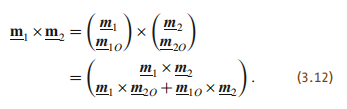第二种是运动和力做叉积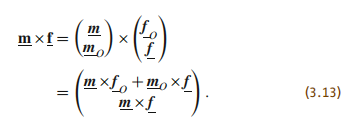在此定义一个叉积算子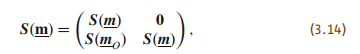所以运动和运动的叉积表示为m$_1$×m$_2$=S(m$_1$)m$_2$
但是运动和力的叉积表示为m×f= - S(m)$^T$f
可见S(m)将运动向量映射为运动向量，S(m)$^T$将力向量映射为力向量。
7、微分
六维空间向量的微分定义为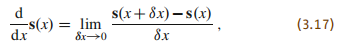对于一个运动的坐标系$A$，任意的六维空间向量s，该空间向量的微分表示为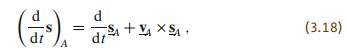且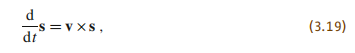8、加速度
六维空间向量的加速度和经典的刚体的加速度是不一样的。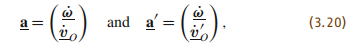上式中，左式为空间向量的加速度，右式为经典的刚体加速度，两者之间存在如下关系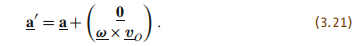如果r是某一个刚体相对于任意一个固定点的位置向量，那么存在如下关系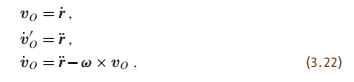上式中，第一个式子是刚体的速度，第二个式子是刚体的经典加速度，第三个式子是刚体在向量空间的加速度，可见式子(3.21)依然是成立的。
如果刚体$B_1$和刚体$B_2$的速度分别是v$_1$和v$_2$，刚体$B_1$相对于刚体$B_2$的速度为v$rel$，那么v$_2$=v$_1$+v$_{rel}$
他们之间的加速度满足如下关系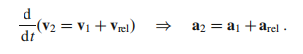9、空间动量
假设一个刚体的质量为$m$，质心为C，绕过C的直线的转动惯量为$I^{cm}$。如果刚体的空间速度为v$_c=(w^Tv_c^T)^T$，那么
线动量为$h=mv_c$
角动量为$h_C=I^{cm}w$
该刚体绕着某一个点$O$的动量为$h_O=h_C+c×h$，其中，$c=\vec{OC}$，即存在如下关系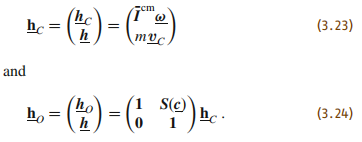10、空间惯量
刚体的空间动量是其空间惯量和速度的点积h=$Iv$。
用在C处的普吕克坐标来表示为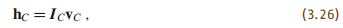其中，上式是对一个质心在C处的刚体的空间惯量的一般表示方式。
但是对于另一点O来说，该刚体的动量表示为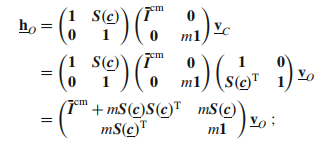但是该式仍然满足h$_C$=$I_Cv_C$的形式，所以可得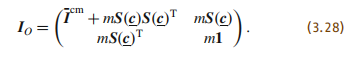我们继而写成如下形式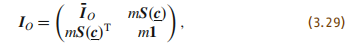其中，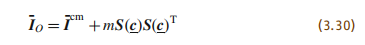空间惯量矩阵是对称矩阵，也是正定矩阵。要表示空间惯量矩阵需要21个变量，但是刚体的惯量矩阵实际上只有10个参数：质量(1)、质心坐标(3)、$I_O$或者$I^{cm}$的六个独立参数(6)。
对于不同的坐标系$A$和$B$，惯量矩阵之间的变换矩阵为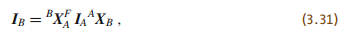故而下式也成立。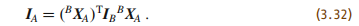如果两个刚体的惯量分别是$I_1$和$i_2$，务必注意他俩相对于同一个旋转轴，那么总惯量为$I_{tot}=I_1+I_2$，
其动能为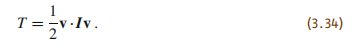11、空间运动方程
刚体的受力等于其动量的变化率，故而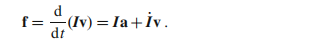再故而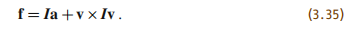展开全文线性代数 矩阵 抽象代数
• 目录： 一、向量的内积和几何意义 二、向量的外积和几何意义 ...两个向量的外积，又叫向量积、叉乘等。外积的运算结果是一个向量而不是一个标量。并且两个向量的叉积与这两个向量组成的坐标平面垂直。 对于向量...
目录：

一、向量的内积和几何意义

二、向量的外积和几何意义

一、向量的内积和几何意义（点乘）

对于向量a和向量b：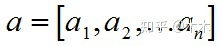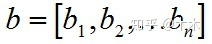1、a和b的内积公式为：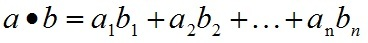要求一维向量a和向量b的行列数相同。

2、内积的几何意义

点乘的几何意义是可以用来表征或计算两个向量之间的夹角，以及在b向量在a向量方向上的投影。

二、向量的外积和几何意义（叉乘）

两个向量的外积，又叫向量积、叉乘等。外积的运算结果是一个向量而不是一个标量。并且两个向量的叉积与这两个向量组成的坐标平面垂直。

对于向量a和向量b：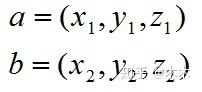1、a和b的外积公式为：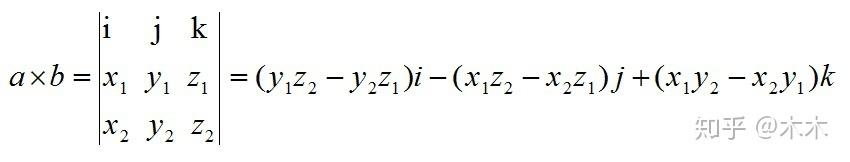其中：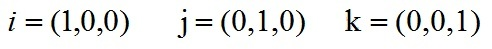根据i、j、k间关系，有：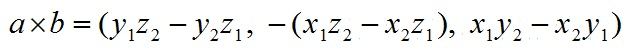2、叉乘几何意义

在三维几何中，向量a和向量b的叉乘结果是一个向量，更为熟知的叫法是法向量，该向量垂直于a和b向量构成的平面。

展开全文• 计算公式 几何意义 两个向量之间的夹角 向量b在向量a上的投影 ...外 ...外在数值上等于两个向量组成平行四边形的面积 reference https://www.cnblogs.com/gxcdream/p/7597865.html ...
• 问题： 已知空间3点的坐标P1(x1,y1,z1),P2(x2,y2,z2),...向量间夹角的余弦等于向量的数量除以向量模的 (LaTex语法公式) \vec{P1P2}=(x 2-x1,y2-y1,z2-z1) \vec{P2P3}=(x3-x2,y2-y1,z2-z1) |\vec{P1P2}|=\sq...PHP
• 本节为线性代数复习笔记的第二部分，矩阵的概念与计算（1），主要包括：向量组的秩，向量、正交、模，施密特标准正交化（正交规范化），向量空间以及坐标变换公式。 1. 向量组的秩   向量组α1⃗,α2⃗,...,...线性代数 数学
• ## 向量

2020-03-24 16:01:42
1.数量积（点乘） 数量积（点乘）的结果是数，用“”·“”来运算，模相乘再...2.向量积（叉乘）： 向量积的定义 性质 向量积满足结合律和分配律 交换律是相反的 向量积的三阶行列式运算 正负正： 数量积和向量...
• 向量的模:向量的大小或长度. 向量与标量向乘:加减速或改变...向量点乘:内.a*b = ax*bx + ay*by.点乘等于向量大小与向量夹角cos的. 向量与矩阵相乘: 坐标坐标系旋转后的矩阵为M: |cos sin| ...
• 中学向量的教学内容除核心内容外，可考虑增加向量积．教材应以学生的心理发展为依据，建立合理的螺旋式体系．对于向量的运算，宜先用几何方法引入，然后推得向量的坐标运算公式，再证明各种运算律．
• 其实,齐次坐标系中三点共线,可以等价于求二维欧几里得坐标系中三个向量共面. 第一种方法是利用公式:a⋅(b×c)=0 \mathbf{a} \cdot(\mathbf{b} \times \mathbf{c})=0 a⋅(b×c)=0 三个向量的混合等于0,就证明它们共...线性代数 算法 c++ 计算机视觉
• 8. 向量的向量积 9. 向量及其坐标的有关公式 二、空间中的曲面与曲线 1. 曲面与曲面方程 2. 空间曲线的方程 3. 旋转面方程 4. 柱面方程 5. 曲线在坐标面上的投影 三、空间中的平面与直线方程 1. 平面方程 2. 直线...线性代数
• 向量内积（点积、数量积）与外积（叉积、向量积） 1.内积 a和b的点积公式为：（也就是投影） a.b=|a||b|cos（a, b） 。 1）推导下面坐标表达式： ...数学基础
• 在笛卡尔坐标系中有向量 (x1,y1)和(x2,y2),他们间夹角的cos值等于 向量 除以 两个向量摸的乘积。 如果你仔细比较一下 求向量夹角的公式和皮尔逊公式,你会发现他们之间很相似！但还是有点不一样。 是的...
• 向量的点,也叫向量的内、数量... Y 1) 坐标点到原点的距离公式为：sqrt( X1^2 + X2^2 + .... + Xn^2)所以可以采用向量表示： sqrt( V. V)，也就是等于向量与自己本身的点再开根号。因此在图形学里，计算坐标3d
• 一、复习几个矩阵的基本知识 1. 向量 1）既有大小又有方向的量成为向量，物理学中...公式：a · b= b · a =|a| * |b| * cosθ = x1 * x2 + y1 * y2点乘又叫向量的内、数量，是一个向量a和它在另一个向量b上的...
• 正常求2个向量夹角用内积公式就可以计算，然而求得的结果不包含方向信息。 如果需要方向信息的话需要引入向量的外来帮助我们判断。 theta是两个向量的夹角，n是垂直与2维平面的方向向量，由右手定则可以判断方向...抽象代数
• n维向量的概念及其线性运算 n维向量的概念 n维向量的线性运算 线性组合和线性表示 向量组的线性相关性 线性相关和线性无关 ...坐标坐标变换公式 向量的内和正交性 标准正交基和Schmidt正交化方法...线性代数
• （结合律，分配律）3）向量分量坐标的方向符合右手系，向量积的定义与此密切相关；4）模：a(a1,a2,..,a3) 的模|a|=(a1^2+a2^2...an^2)^(1/2);5)方向角和方向余弦：这个公式很重要，后面很多定理的证明其实都是基于此...
• 解析几何（一）两个向量的夹角解法 最近在项目中需要判断点和四边形关系，我的算法中涉及...使用向量夹角公式 cos = 两向量 / 两向量模的乘积 = arccos( 两向量 / 两向量模的乘积 )   1 #inclu
• 使用向量夹角公式 cos<夹角> = 两向量 / 两向量模的乘积 <夹角> = arccos( 两向量 / 两向量模的乘积 ) 1 #include <cmath> 2 using std::acos; // 反余弦函数 3 using st...
• 坐标变换公式7.向量的内8.Schmidt正交化9.正交基及规范正交基 1.有关向量组的线性表示 (1)α1,α2,⋯ ,αs\alpha_{1},\alpha_{2},\cdots,\alpha_{s}α1​,α2​,⋯,αs​线性相关⇔\Leftrightarrow⇔至少有一个...线性代数 机器学习
• 一、向量(Vectors) 向量表示示意图： 有序实数组，用以表示在不同...点乘（内，结果为标量，几何意义是 [公式] ，满足分配率和交换律，可用于判断两向量夹角，求向量长度，计算投影等） 叉乘（外，结果为向量...
• 【转载】关于欧拉四面体公式的推导及证明过程 1，建议x，y，z直角坐标系。设A、B、C少拿点的坐标分别为(a1,b,1,c1),(a2,b2,c2),(a3,b3,c3),四面体O-...3，根据矢量数量坐标表达式及数量的定义得 又...
• 文章目录1概述2向量和叉积2.1向量的点2.2 向量的叉积3 旋转和变换3.1 变换矩阵与齐次坐标3.2 旋转向量3.3 四元数4 李群和李代数4.1 群4.2 李代数4.3 李代数求导与扰动模型4.3.1 BCH公式与近似形式4.3.2 李代数...计算机视觉